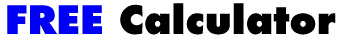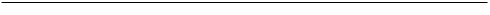FREE Software! Download Free Math Calculator Download (33 kb)A 32 bit Windows console mode math calculator with 26 registers to store values, 19 predefined functions and user defined functions with parameters. Use many built in functions and define your own! Type expressions in natural math syntax! Best of all, it's completely free! Use natural math syntax like: x = 25 y = 300 (10*x + y)/2 275 last*3 825 To make any calculations a snap! Here is the calculator help to show you what's supported: Standard functions: acos asin atan cos sin tan deg rad exp ln log round sqrt abs cosh sinh tanh pi last Operators: +(add) -(subtract, minus) *(multiply) /(divide) %(modulo) ^(exponent) '=' assigns a value to one letter memory register (for later use), e.g. x = sin(30)/(5*log(2) - 2^3) To define user function type: def:name(p0,p1,...,p9)=expression with up to 10 formal parameters named from p0 to p9, examples: def:cot(p0) = 1/tan(p0) or def:const=-15.67 Function name may be from 2 to 6 characters long, you can define up to 20 functions. New functions can reference previous functions. To undefine type: undef:name You can use upper or lower case in everything. Numbers can use exponential notation, eg. 1.6e-6 Type an expression and press , or use / followed by one letter command: /d set angle unit to Degrees /q Quit (or press Ctrl-C) (default at start) /r set angle unit to Radians /h to see this Help /s Show memory registers /f display User Defined Functions /c clear all functions & regs. /v saVe /l load /pnn set decimal precision Return to the Hyperionics Home Page.